# How to develop algorithmic thinking in data structure and algorithms?

Algorithmic Thinking has recently become a buzzword among programmers. It is a method for solving problems based on a clear definition of the steps: logically and repeatedly. The best idea would be to develop this skill independently from learning programming, where proper visualisation can help us understand several efficient problem-solving approaches.

### Steps of algorithmic thinking

Step1 : Comprehending the problem statement

• Do we understand every word used within the problem?
• What data or information are provided as input?
• What data or results are requested as an output?
• Understanding and extracting computational and non-computational details relevant to the problem — data structures, particular instances of the problem, the general cases of the problem, critical operations, the specific constraint on input, distribution of the input, mathematics, physics, etc.
• Describe the solution qualitatively, i.e., using your own words or drawing a clear visualisation.

Step 2 : Selecting concepts that can be applied

• Making some predictions regarding the concepts based only upon problem description and list down all theoretical concepts.
• Have we solved any similar problems? If yes, then take advantage of that experience. Identify already known algorithms of similar problems.
• Are we going to use all the information available in the problem statement? Is it possible to eliminate unnecessary data?
• Selecting a structure to simplify data handling: function, arrays, linked lists, loop, stack, queue, hash table, local variables, global variables, etc.

Step 3 :  Formulation of an algorithm strategy

• Exploring the hand-written way to solve the problem by going through several examples, then attempt to create a generalisation. To do this, carefully think about each step and observe what actions are common to every example.
• Identifying the solution strategy based on the nature of the problem like divide and conquer, dynamic programming, backtracking, greedy, using a data structure, using numbers theory, etc. Two types of thinking

Iterative Thinking — solving the larger problem by building the partial solution incrementally.

Recursive Thinking — solving the larger problem using the solution of the smaller problem.

• Designing and writing step-by-step solutions in simple English
• Visualising solution on the paper by describing operations to transform given input to the desired output.
• Transforming the description into pseudocode or flowchart.
• Transforming the pseudocode into a working code using your favorite programming language. Take care of the correct coding style.

Step 4 : Verifying correctness and optimising further

• Manually analysing the input-output pattern by performing a hand-trace and, if needed, drawing a plot to describe the behavior of the variables or critical steps defined in the algorithm.
• Is there some input for which our algorithm is not providing correct output? Does it work for all boundary cases?
• If there is an error or bug — identifying the steps which are responsible for that? How to correct that bug or error?
• After verifying correctness, now we should analyse the efficiency by counting the total number of operations with respect to the input size(n). Sometimes we need to define the abstract mathematical model to calculate the algorithms’ steps like recurrence, summation, etc.
• Now the critical step is to optimize the solution and solving it using a fewer number of steps. But how we do it? We can identify some essential details of the problem to try a different problem-solving approach. We should keep optimising the solution further until there is no further possibility.
• Sometimes we can also optimize the solution by reducing some intermediate steps in the pseudocode.

### Algorithmic thinking via solving puzzles

Solving algorithmic puzzles is a most productive and enjoyable way to develop algorithmic thinking skills. Puzzles are an ideal vehicle for mastering this skill for three reasons —

• Solving puzzles are always fun where a learner is normally willing to put more effort into solving them than everyday professional work.
• Most of us have a natural tendency to think about computer language problems instead of applying problem-solving strategies. Here algorithmic puzzles help us think on a more abstract level to develop ideas related to problem-solving strategies.
• Sometimes interviewers offering problems during interviews claim that they are more interested in how an interviewee approaches a problem than it's the implementation of the solution. Showing expertise in applying problem-solving strategies and analysis can be a highly attractive way to impress the potential employer.

But before moving forward, we would like to make an essential comment on different types of algorithmic puzzles —

• Every puzzle has an input that defines an instance of the puzzle. The instance can be either specific (e.g., find a false coin among eight coins with a balance) or general (e.g., find a false coin among N coins with a balance).
• When dealing with a specific instance of a puzzle, the learner has to focus on the particular instance given. It might be the case that other instances of the puzzle do not have the same solution or even do not have solutions at all!
• On the other hand, solving the general instance of the puzzle could be more satisfying and even more comfortable most of the time.
• But whether a puzzle is presented by a specific instance or given in its most general form, it is almost always a good idea to solve a few small instances of it anyway because it can provide useful insights into the puzzle given.

Best puzzles to warm up algorithmic thinking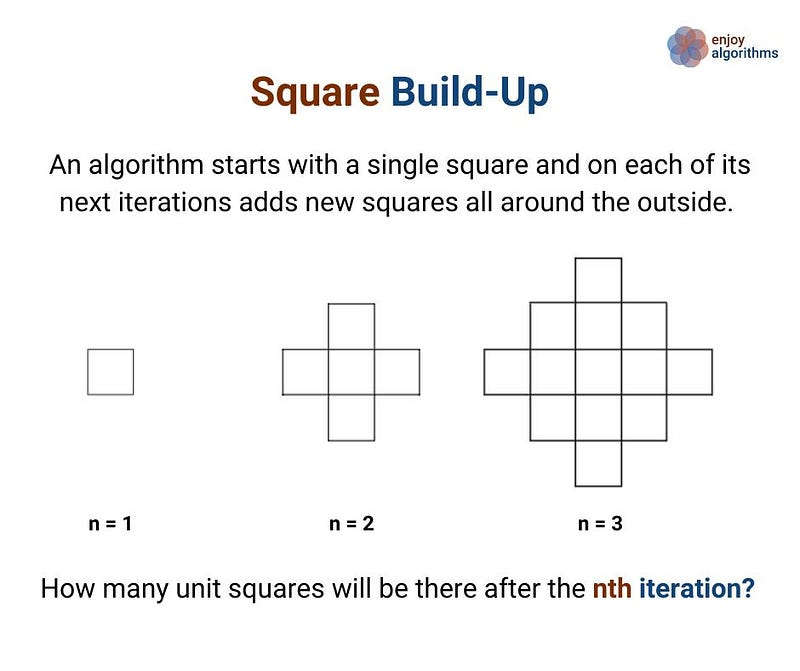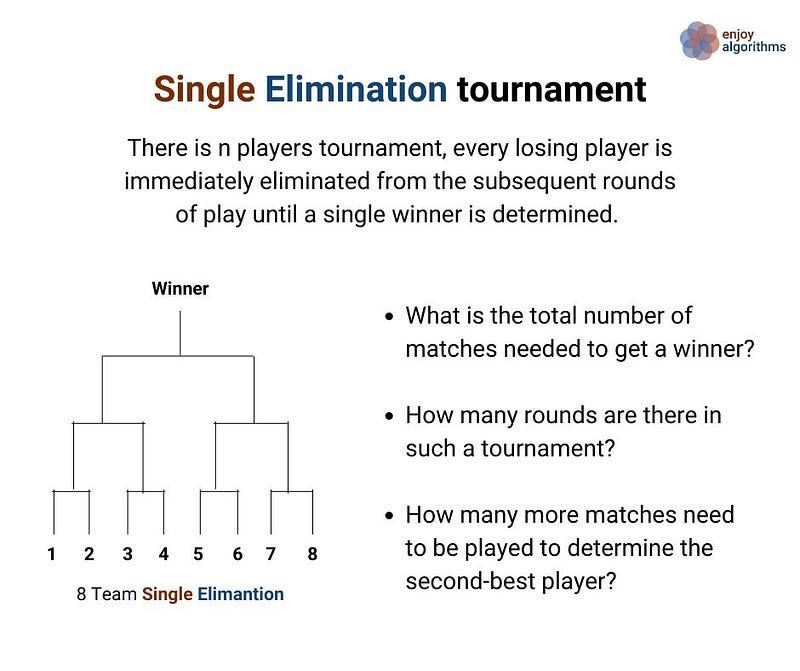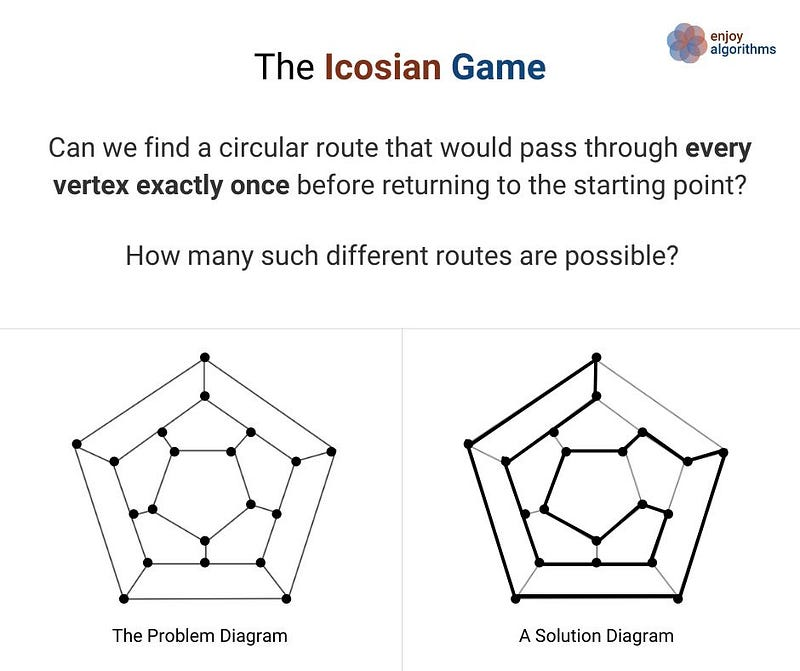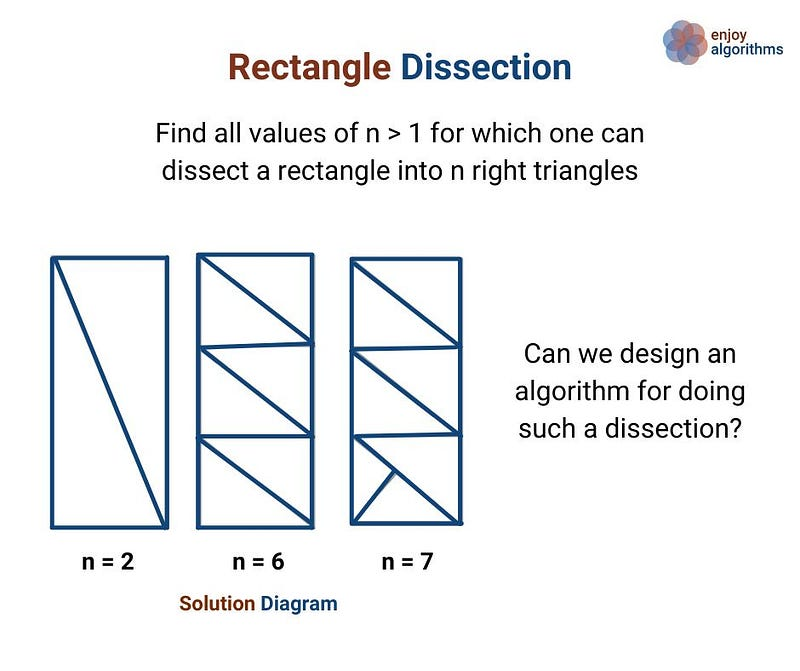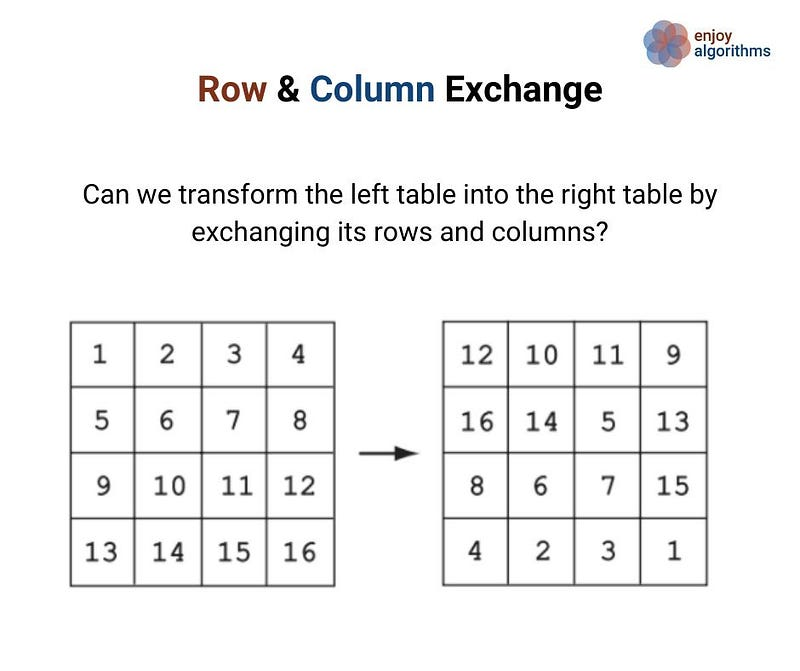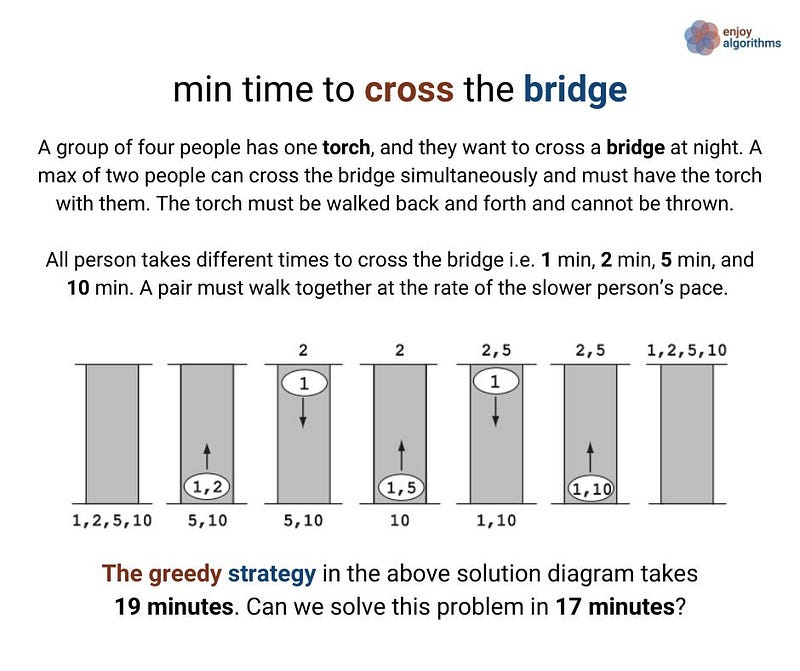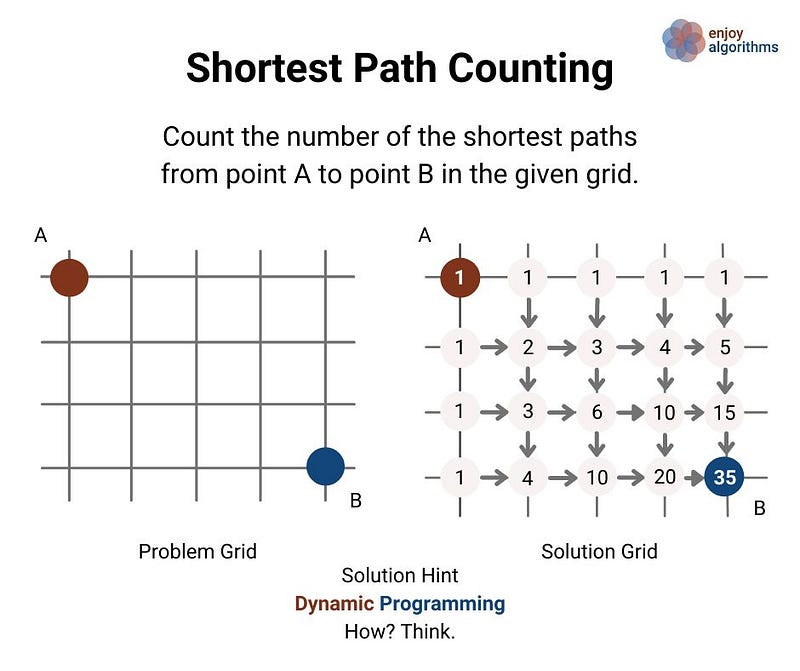###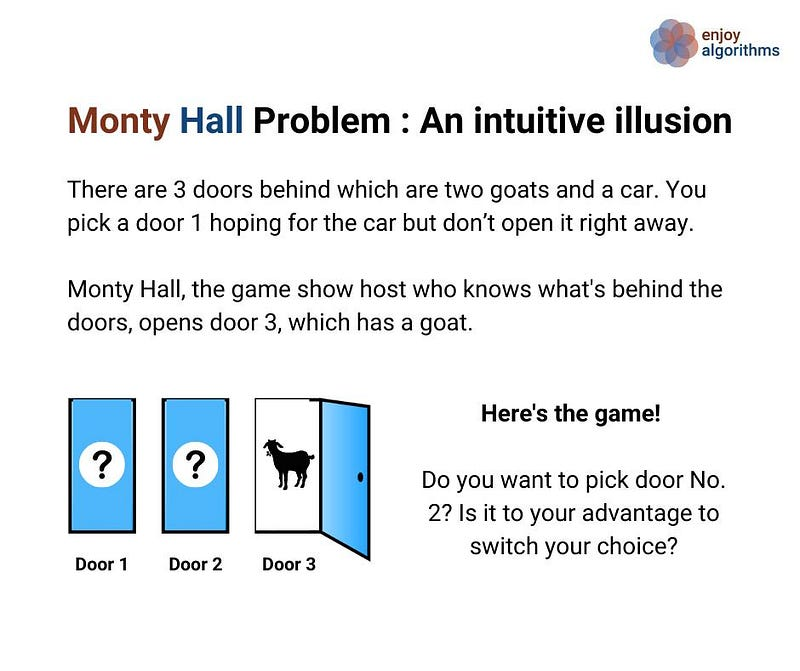Problem solving strategies in algorithms

The purpose of this section is to highlight some general strategies for designing algorithms and solving problems in computer science. Learning to apply these strategies could be one of the best achievements for the learners.

1. Incremental approach

Examples: Insertion Sort, Finding max and min in an array

One of the simple ideas of our daily problem-solving activities where we build the solution partially step by step. There is a different variation to it:

• Input-centric strategy — At each step of the iteration, we process one input and build the partial solution.
• Output-centric strategy — At each step of the iteration, we add one output to the solution and build the partial solution.
• Iterative improvement strategy — Here, we start with some easily available approximation to a solution and continuously improves upon it to reach the final solution.

2. Decrease and conquer approach

Examples: Euclid algorithm of finding GCD, Josephus problem

The decrease-and-conquer strategy is based on finding the solution to a given problem via its one sub-problem solution. Such an approach leads naturally to a recursive algorithm, which reduces the problem to a sequence of smaller input size. Until it becomes small enough to be solved, i.e., it reaches the recursion’s base case.

3. Divide and conquer approach

Examples: Merge Sort, Quick Sort

The divide-and-conquer strategy is to partition a problem into more than one subproblems, solve each of them, and then, if necessary, combine their solutions to get a solution to the original problem. We solve many fundamental problems efficiently in computer science by using this strategy.

4. Transform and conquer approach

Examples: Many problems based on array or list are easy to solve if array or list are sorted i.e. Pre-sorting based algorithms (Finding closest pair of points, check whether all the elements in given array are distinct, etc)

The transform-and-conquer is a problem-solving approach that is based on the idea of transformation. A problem is solved in two stages:

1. Transformation stage : We transform the original problem into another problem that is easier to solve.
2. Conquering stage : Now, we solve the transformed problem.

We can identify three varieties of this problem-solving strategy:

• Instance simplification strategy — transforming an instance of the given problem into another instance of the same problem with some special property that makes the problem easier to solve.
• Representation change strategy — Based on the transformation of a problem’s input to a different representation that is more conducive to an efficient algorithmic solution.
• Problem reduction strategy — Here instance of a given problem is transformed into an instance of a different problem altogether.

5. Greedy approach

Examples: Fractional Knapsack, Dijkstra algorithm of shortest path

The greedy approach solves an optimisation problem by expanding a partially constructed solution until a complete solution is reached. At each step, we take a greedy choice and add it to the partially constructed solution. This idea produces the optimal global solution without violating the problem’s constraints.

• The greedy choice is the best alternative available at each step is made in the hope that a sequence of locally optimal choices will yield a (globally) optimal solution to the entire problem.
• This approach works in some cases but fails in others. Usually, it is not difficult to design a greedy algorithm itself, but a more difficult task is to prove that it produces an optimal solution.

6. Dynamic programming

Examples: Finding nth fibonacci, longest common subsequence

Dynamic programming is one of the most popular techniques for solving problems with overlapping or repeated subproblems. This

• Rather than solving overlapping subproblems, again and again, we solve each smaller subproblems only once and store the results in memory.
• We solve a lot of optimisation and counting problems using the idea of dynamic programming.

7. Exhaustive search

Examples: find all permutation of a string, Print all subset

An exhaustive search can solve many problems — a strategy that explores all possibilities of solutions until a solution to the problem is found. Therefore, problems are rarely offered to a person to solve the problem using this strategy.

The most crucial limitation of exhaustive search is its inefficiency: as a rule, the number of solution candidates that need to be processed grows at least exponentially with the problem size, making the approach inappropriate not only for a human but often for a computer as well.

8. Backtracking

Example: Rat in a maze, N-queen problem

Backtracking is an improvement over the approach of exhaustive search. It is a method for generating a solution by avoiding unnecessary possibilities of the solutions! The main idea is to build a solution one piece at a time and evaluate each partial solution as follows:

• If a partial solution can be developed further without violating the problem’s constraints, then it is done by taking the first remaining valid option at the next stage. (Think!)
• Suppose there is no valid option at the next stage, i.e. If there is a violation of the problem constraint, the algorithm backtracks to replace the partial solution’s previous stage with the following option for that stage. (Think!)

In simple words, backtracking involves undoing several wrong choices — the smaller this number, the faster the algorithm finds a solution. In the worst-case scenario, a backtracking algorithm may end up generating all the solutions as an exhaustive search, but this rarely happens!

Source: Algorithmic Puzzles by Anany Levitin and Maria Levitin

Enjoy learning, Enjoy coding, Enjoy algorithms!

Get well-designed application and interview centirc content on ds-algorithms, machine learning, system design and oops. Content will be delivered weekly.